×#### Thank you for registering.

One of our academic counsellors will contact you within 1 working day.

Click to Chat

1800-1023-196

+91-120-4616500

CART 0

• 0

MY CART (5)

Use Coupon: CART20 and get 20% off on all online Study Material

ITEM
DETAILS
MRP
DISCOUNT
FINAL PRICE
Total Price: Rs.

There are no items in this cart.
Continue Shopping```Integration By PartsAs discussed in the previous sections, while attempting to compute integrals of functions, we may either use substitution method, partial fractions or integrate the function using by parts. While using integration by parts, you just need to remember a simple formula and apply the same. But, care is to be taken in the selection of the first and the second functions.

If u and v be two differentiable functions of x, then the differential of the product uv is found by

d(uv) = u dv + v du

Integral of the product of these two functions is given by: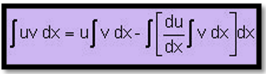Example:

Let us try to integrate the function x cos x.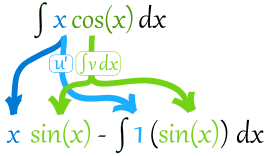Note:

In applying the above rule, care has to be taken in the selection of the first function (u) and the second function (v). Normally we use the following methods:If in the product of the two functions, one of the functions is not directly integrable

(e.g. ln x, sin-1x, cos-1x, tan-1x etc.) then we take it as the first function and the remaining function is taken as the second function. e.g. In the integration of ∫ tan-1x dx, tan-1x is taken as the first function and x as the second function.

If there is no other function, then unity is taken as the second function e.g. In the integration of ∫ tan-1x dx , tan-1x is taken as the first function and 1 as the second function.

If both of the functions are directly integrable then the first function is chosen in such a way that the derivative of the function thus obtained under integral sign is easily integrable.

The chart given below illustrates the preference order generally adopted for the selection of the first function: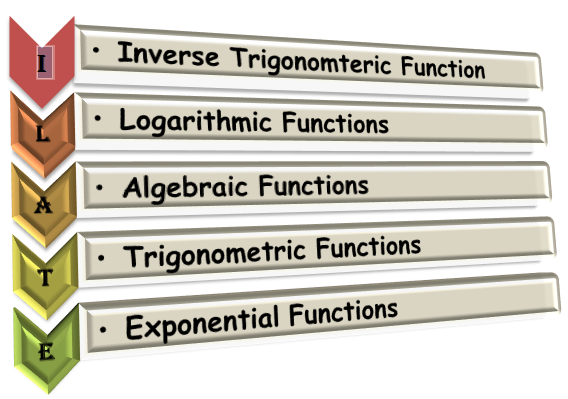(Inverse, Logarithmic, Algebraic, Trigonometric, Exponent)

In the above stated order, the function on the left is always chosen as the first function. This rule is called as ILATE.  e.g. In the integration of ∫ x sin x,  x is taken as the first function and sin x is taken as the second function.

Watch this Video for more reference

Example 1:

Evaluate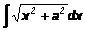.

Solution: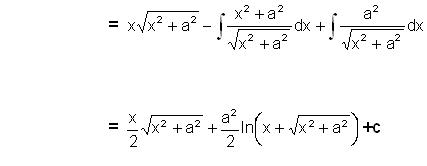____________________________________________________________________________

Example 2:

Evaluate ∫ sec3θ dθ.

Solution:

I = ∫ sec3 θ dθ

= sec θ ∫ sec2 θ dθ – ∫ tan θ (sec θ tan θ) dθ

= sec θ tan θ – ∫(sec2 θ – 1) sec θ dθ

= sec θ tan θ – I + ln |sec θ + tan θ|

⇒ I = 1/2 [sec θ tan θ] + 1/2 In |sec θ tan θ| + c

___________________________________________________________________________

Example 3:

Evaluate ∫ x3 In x dx.

Solution:

Here u = ln x ⇒ du = 1/x dx

and v = x3 ⇒ dv = 3x2 dx

I = 1/4 x4 In x – 1/4 x4 In x – 1/16 x4 + c

= x4/4 .In x – 1/4 ∫ x3 dx = 1/4 x4 In x – 1/6 x4 + c.Short Method:

When one function is xn, n ∈ N, then the result in such a case is

∫ xn v dx =  xn ∫ v dx – F(x), where F(x) is obtained by applying the rule (derivative of first) . (integral of second) on every function obtained previously and writing them in alternate sign.

In the integral ∫g(x) ex dx, if g(x) can be expressed as g(x) = f(x) + f'(x) then = ∫ ex [f(x) + f(x)] dx = ex f(x) + c.

In the integral ∫ [xf'(x) + f(x)] dx, the direct result which can be used is  ∫ [xf'(x) + f(x)] dx =  x f(x) + c.

Example 4:

Evaluate ∫ ex (1/x – 1/x2)dx.

Solution:

Let I = ∫ ex (1/x – 1/x2)dx

Here f(x) = 1/x, f'(x) = –1/x2

Hence I = ex / x + cQ1. While integrating by parts,

(a) any function can be assumed to be the first or the second.

(b) the function which can’t be integrated easily should be assumed to be the second function.

(c) the function which can be integrated easily should be assumed as the first function.

(d) the function which can be integrated easily should be assumed as the second function.

Q2. When one of the function is xn, then  ∫ xn v dx =

(a) xn ∫v dx –  ∫v dx

(b)  ∫ xn v dx = xn ∫v dx – F(x), where F(x) can be computed using an appropriate rule.

(c) any function can be assumed to be the first or the second and then the integral can be found using the long method.

(d) none of these.

Q3. While integrating the product of an algebraic and an exponential function,

(a) algebraic function should be assumed as the first function and exponential as the second.

(b) any function can be assumed to be the first or the second.

(c) exponential function should be assumed as the first function and algebraic as the second.

(d) order is not really required.

Q4. While integrating by parts, the rule to be followed is

(a) TIALE

(b) LAITE

(c) ILATE

(d) TAILE

Q5. The integral of the product of two functions a and b is given by

(a) ∫ ab dx = a ∫ b dx – ∫ [da/dx ∫ b dx ] dx

(b)  ∫ ab dx = b ∫ a dx – ∫ [da/dx ∫ b dx ] dx

(c)  ∫ ab dx = a ∫ b dx – ∫ [db/dx ∫ a dx ] dx

(d)  ∫ ab dx = b ∫ a dx – ∫ [db/dx ∫ a dx ] dx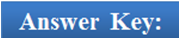Q1.

Q2.

Q3.

Q4.

Q5.

(d)

(b)

(a)

(c)

(a)

Related Resources

You may also refer Integration by Partial Fractions.

For getting an idea of the type of questions asked, refer the previous year papers.

To read more, Buy study materials of Indefinite integral comprising study notes, revision notes, video lectures, previous year solved questions etc. Also browse for more study materials on Mathematics here.
```### Course Features

• 731 Video Lectures
• Revision Notes
• Previous Year Papers
• Mind Map
• Study Planner
• NCERT Solutions
• Discussion Forum
• Test paper with Video Solution